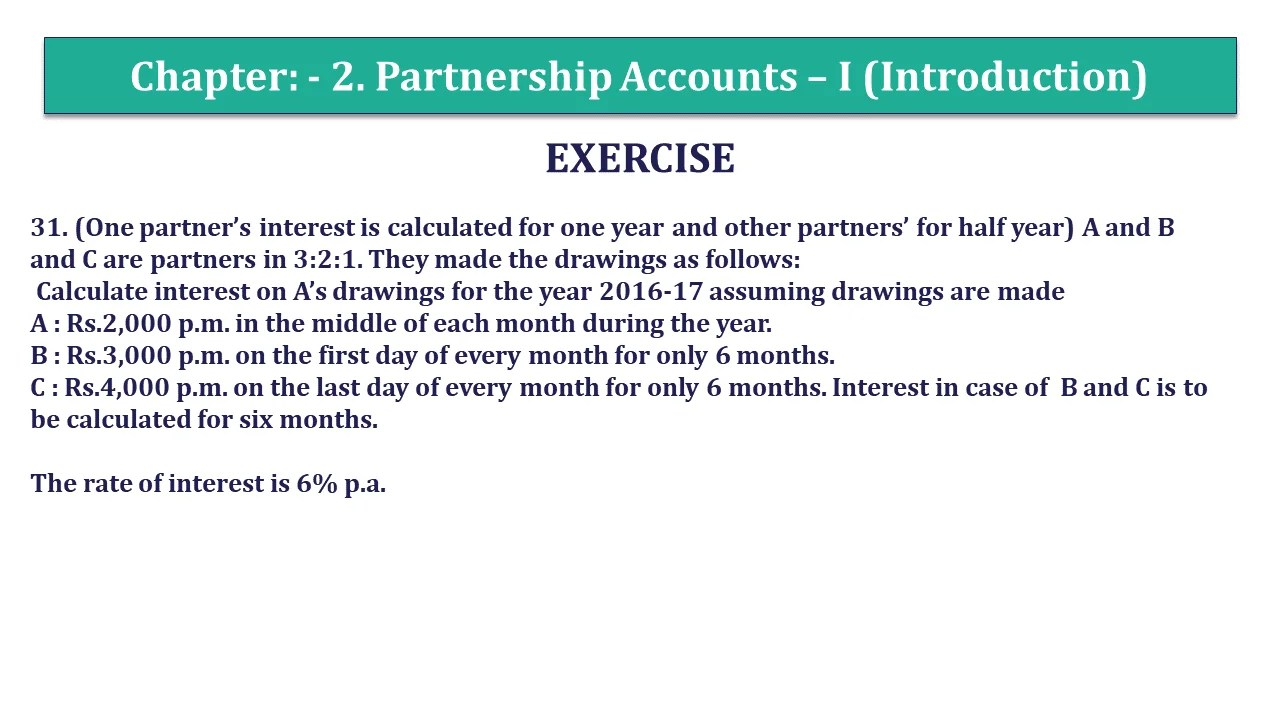# Question 31 Chapter 2 of +2 Part-1 – USHA Publication 12 Class Part – 1Question 31 Chapter 2 of +2-Part-1

31. (One partner’s interest is calculated for one year and other partners’ for half year) A and B and C are partners in 3:2:1. They made the drawings as follows:
Calculate interest on A’s drawings for the year 2016-17 assuming drawings are made
A : Rs.2,000 p.m. in the middle of each month during the year.
B : Rs.3,000 p.m. on the first day of every month for only 6 months.
C : Rs.4,000 p.m. on the last day of every month for only 6 months. Interest in case of B and C is to be calculated for six months.
The rate of interest is 6% p.a.

### The solution of Question 31 Chapter 2 of +2 Part-1: –

Calculation of interest on partner’s Drawings
If partners withdrawal the same amount of drawing on a regular basis then we can calculate the interest on drawing with help of following formula: –
Interest on Drawing = Total Drawings X Rate of Interest X Period/12 Months
Rate of Interest = 6%
Period = the period/time will be calculated with the help of following formula: –

 Period = Time left after 1st withdrawal + Time left after Last withdrawal 2

A: Rs.2,000 p.m. in the middle of each month during the year:

A’s withdrawal at the middle of every month = 2,000 p.m. Therefore, total drawings of the year= Rs.24,000.
It means 1st withdrawal has made on 15-04-20.
So, Time left from 15-4-20.. to 1-3-20.. is 11 months and 15 days. = 11.5 months
And last withdrawal has made on 15-3-20.
So, Time left from 15-3-20.. to 31-3-20. is 15 days = 0.5 month

Now, put this time period in the formula

 Period = 11.5 + 0.5 2
 Period = 12 2
 Period = 6

Total Drawings = 2,000*12=24,000

 Interest on Drawing = 24,000 X 6 X 6 100 12 Interest on Drawing = 720/-

B: Rs.3,000 p.m. on the first day of every month for only 6 months.

B’s withdrawal at the beginning of every month = 3,000 p.m. Therefore, total drawings of the year= Rs.18,000.
It means 1st withdrawal has made on 01-04-20.
So, Time left from 1-4-20… to 1-3-20… is 6 Months
And last withdrawal has made on 1-3-20.
So, Time left from 1-3-20… to 31-3-20… is 1 month.

Now, put this time period in the formula:

 Period = 6 + 1 2
 Period = 7 2
 Period = 3.5

Total Drawings = 3,000*6 = 18,000

 Interest on Drawing = 18,000 X 6 X 3.5 100 12 Interest on Drawing = 315/-

C: Rs.4,000 p.m. on the last day of every month for only 6 months.

A’s withdrawal at the end of every month = 4,000 p.m. Therefore, total drawings of the year= Rs.4,000*6 = Rs.24,000
It means 1st withdrawal has made on 31-04-2.
So, Time left from 31-4-20… to 31-9-20… is 5 Months
And last withdrawal has made on 1-9-20.
So, Time left from 31-9-20… to 31-9-20… is 0 month.

 Period = 5 + 0 2
 Period = 5 2
 Period = 2.5
 Interest on Drawing = 24,000 X 6 X 2.5 100 12 Interest on Drawing = 300/-